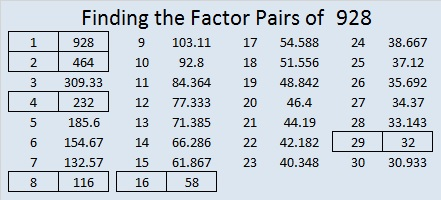# 928 Halloween Cat

This cat has arrived just in time for Halloween. Find the factors that go with the clues in the grid to make this Halloween Cat puzzle a multiplication table:Print the puzzles or type the solution on this excel file: 12 factors 923-931

Now let me tell you a little about the number 928.

It is the sum of four consecutive prime numbers:
227 + 229 + 233 + 239 = 928
and the sum of eight other consecutive prime numbers:
101 + 103 + 107 + 109 + 113 + 127 + 131 + 137 = 928

It is the sum of two squares:
28² + 12² = 928
That means 928 is the hypotenuse of a Pythagorean triple:
640-672-928 which is 28² – 12², 2(28)(12), 28² + 12²

Here’s how 928 looks in a few other bases:
It’s 565 in BASE 13, because 5(169) + 6(13) + 5(1) = 928.
It’s 4A4 in BASE 14 (A is 10 base 10), because 4(196) + 10(14) + 4(1) = 928.
It’s TT in BASE 31 (T is 29 base 10), because 29(31) + 29(1) = 29(32) = 928.
It’s T0 in BASE 32, because 29(32) = 928.

• 928 is a composite number.
• Prime factorization: 928 = 2 × 2 × 2 × 2 × 2 × 29, which can be written 732 = 2⁵ × 29
• The exponents in the prime factorization are 5 and 1. Adding one to each and multiplying we get (5 + 1)(1 + 1) = 6 × 2 = 12. Therefore 928 has exactly 12 factors.
• Factors of 928: 1, 2, 4, 8, 16, 29, 32, 58, 116, 232, 464, 928
• Factor pairs: 928 = 1 × 928, 2 × 464, 4 × 232, 8 × 116, 16 × 58, or 29 × 32
• Taking the factor pair with the largest square number factor, we get √928 = (√16)(√58) = 4√58 ≈ 30.463092423.This site uses Akismet to reduce spam. Learn how your comment data is processed.